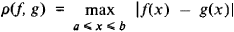# complete metric space

(redirected from Completeness (topology))

## complete metric space

[kəm′plēt ¦me·trik ′spās]
(mathematics)
A metric space in which every Cauchy sequence converges to a point of the space. Also known as complete space.
McGraw-Hill Dictionary of Scientific & Technical Terms, 6E, Copyright © 2003 by The McGraw-Hill Companies, Inc.
The following article is from The Great Soviet Encyclopedia (1979). It might be outdated or ideologically biased.

## Complete Metric Space

a metric space in which the Cauchy convergence criterion is satisfied.

A sequence of points x1, x2, …, xn, … on a line, in a plane, or in space is said to be a Cauchy, or fundamental, sequence if for sufficiently large numbers n and m the distance between the points xn and xm becomes arbitrarily small. According to the Cauchy criterion, such a sequence of points has a limit if, and only if, it is a Cauchy sequence. For many sets of mathematical objects, such as functions or operators, a concept of distance can be introduced that has properties analogous to the properties of ordinary distance. The set is then said to be a metric space. The concept of the limit of a sequence of points in the metric space can be defined in the usual way. If the Cauchy criterion holds, the space is called complete.

Examples of complete metric spaces are Euclidean spaces and many other linear spaces—for example, the space of continuous functions on the interval [a, b] with distanceand Hubert space. A closed subset of a complete metric space is itself a complete metric space. If a metric space is not complete, it can be made complete in a way analogous to the way the set of rational numbers can be augmented by the irrationals to form the set of real numbers.

The concept of completeness can be extended to nonmetric topological spaces in which neighborhoods of different points can be compared. Examples of such spaces are topological groups and rings.